sortClasses

Sort classes of confusion matrix chart

Description

example

sortClasses(cm,order) sorts the classes of the confusion matrix chart cm in the order specified by order. You can sort the classes in their natural order, by the values along the diagonal of the confusion matrix, or in fixed order that you specify.

Examples

collapse all

Load a sample of predicted and true labels for a classification problem. trueLabels are the true labels for an image classification problem and predictedLabels are the predictions of a convolutional neural network. Create a confusion matrix chart.

figure
cm = confusionchart(trueLabels,predictedLabels);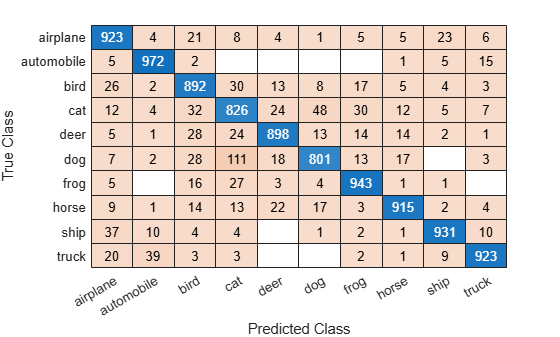Reorder the classes of the confusion matrix chart so that the classes are in a fixed order.

sortClasses(cm, ...
["cat" "dog" "horse" "deer" "bird" "frog", ...
"airplane" "ship" "automobile" "truck"])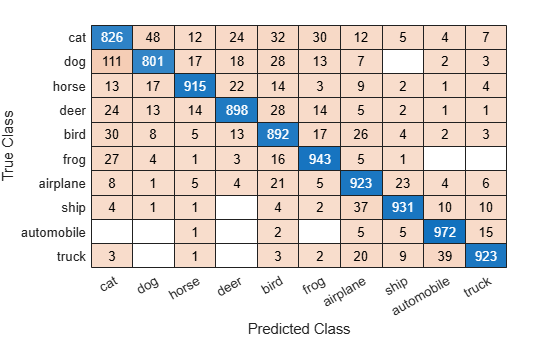Load a sample of predicted and true labels for a classification problem. trueLabels are the true labels for an image classification problem and predictedLabels are the predictions of a convolutional neural network. Create a confusion matrix chart with column and row summaries

figure
cm = confusionchart(trueLabels,predictedLabels, ...
'ColumnSummary','column-normalized', ...
'RowSummary','row-normalized');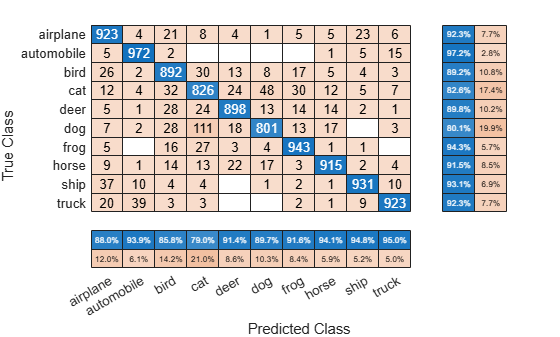To sort the classes of the confusion matrix by class-wise recall (true positive rate), normalize the cell values across each row, that is, by the number of observations that have the same true class. Sort the classes by the corresponding diagonal cell values and reset the normalization of the cell values. The classes are now sorted such that the percentages in the blue cells in the row summaries to the right are decreasing.

cm.Normalization = 'row-normalized';
sortClasses(cm,'descending-diagonal');
cm.Normalization = 'absolute';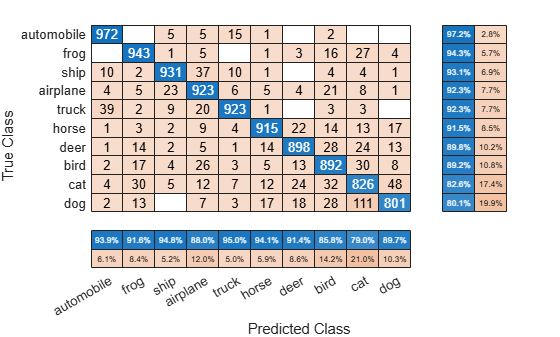To sort the classes by class-wise precision (positive predictive value), normalize the cell values across each column, that is, by the number of observations that have the same predicted class. Sort the classes by the corresponding diagonal cell values and reset the normalization of the cell values. The classes are now sorted such that the percentages in the blue cells in the column summaries at the bottom are decreasing.

cm.Normalization = 'column-normalized';
sortClasses(cm,'descending-diagonal');
cm.Normalization = 'absolute';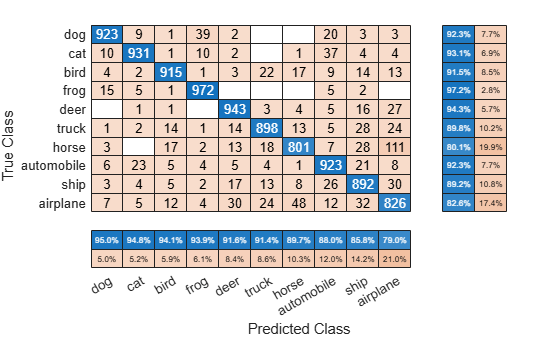Input Arguments

collapse all

Confusion matrix chart, specified as a ConfusionMatrixChart object. To create a confusion matrix chart, use confusionchart,

Order in which to sort the classes of the confusion matrix chart, specified as one of these values:

• 'auto' — Sorts the classes into their natural order as defined by the sort function. For example, if the class labels of the confusion matrix chart are a string vector, then sort alphabetically. If the class labels are an ordinal categorical vector, then use the order of the class labels.

• 'ascending-diagonal' — Sort the classes so that the values along the diagonal of the confusion matrix increase from top left to bottom right.

• 'descending-diagonal' — Sort the classes so that the values along the diagonal of the confusion matrix decrease from top left to bottom right.

• 'cluster' (Requires Statistics and Machine Learning Toolbox™) — Sort the classes to cluster similar classes. You can customize clustering by using the pdist (Statistics and Machine Learning Toolbox), linkage (Statistics and Machine Learning Toolbox), and optimalleaforder (Statistics and Machine Learning Toolbox) functions. For details, see Sort Classes to Cluster Similar Classes (Statistics and Machine Learning Toolbox).

• Array — Sort the classes in a unique order specified by a categorical vector, numeric vector, string vector, character array, cell array of character vectors, or logical vector. The array must be a permutation of the ClassLabels property of the confusion matrix chart.

Example: sortClasses(cm,'ascending-diagonal')